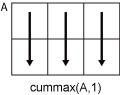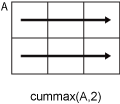Main Content

# cummax

Cumulative maximum

## Syntax

``M = cummax(A)``
``M = cummax(A,dim)``
``M = cummax(___,direction)``
``M = cummax(___,nanflag)``

## Description

example

````M = cummax(A)` returns the cumulative maximum elements of `A`. By default, `cummax(A)` operates along the first array dimension whose size does not equal 1.If `A` is a vector, then `cummax(A)` returns a vector of the same size containing the cumulative maxima of `A`.If `A` is a matrix, then `cummax(A)` returns a matrix of the same size containing the cumulative maxima in each column of `A`.If `A` is a multidimensional array, then `cummax(A)` returns an array of the same size containing the cumulative maxima along the first array dimension of `A` whose size does not equal 1.```

example

````M = cummax(A,dim)` returns the cumulative maxima along the dimension `dim`. For example, if `A` is a matrix, then `cummax(A,2)` returns the cumulative maxima along the rows of `A`.```

example

````M = cummax(___,direction)` optionally specifies the direction using any of the previous syntaxes. You must specify `A` and, optionally, can specify `dim`. For instance, `cummax(A,2,'reverse')` returns the cumulative maxima of `A` by working from end to beginning of the second dimension of `A`.```

example

````M = cummax(___,nanflag)` specifies whether to include or omit `NaN` values from the calculation for any of the previous syntaxes. `cummax(A,'includenan')` includes all `NaN` values in the calculation while `cummax(A,'omitnan')` ignores them.```

## Examples

collapse all

Find the cumulative maxima of a 1-by-10 vector of random integers.

`v = randi(10,1,10)`
```v = 1×10 9 10 2 10 7 1 3 6 10 10 ```
`M = cummax(v)`
```M = 1×10 9 10 10 10 10 10 10 10 10 10 ```

Find the cumulative maxima of the columns of a 3-by-3 matrix.

`A = [3 5 2; 1 6 3; 7 8 1]`
```A = 3×3 3 5 2 1 6 3 7 8 1 ```
`M = cummax(A)`
```M = 3×3 3 5 2 3 6 3 7 8 3 ```

Find the cumulative maxima of the rows of a 3-by-3 matrix.

`A = [3 5 2; 1 6 3; 7 8 1]`
```A = 3×3 3 5 2 1 6 3 7 8 1 ```
`M = cummax(A,2)`
```M = 3×3 3 5 5 1 6 6 7 8 8 ```

Calculate the cumulative maxima in the third dimension of a 2-by-2-by-3 array. Specify `direction` as `'reverse'` to work from the end of the third dimension to the beginning.

`A = cat(3,[1 2; 3 4],[9 10; 11 12],[5 6; 7 8])`
```A = A(:,:,1) = 1 2 3 4 A(:,:,2) = 9 10 11 12 A(:,:,3) = 5 6 7 8 ```
`M = cummax(A,3,'reverse')`
```M = M(:,:,1) = 9 10 11 12 M(:,:,2) = 9 10 11 12 M(:,:,3) = 5 6 7 8 ```

Create a vector containing `NaN` values and compute the cumulative maxima. By default, `cummax` ignores `NaN` values.

```A = [3 5 NaN 9 0 NaN]; M = cummax(A)```
```M = 1×6 3 5 5 9 9 9 ```

If you include `NaN` values in the calculation, then the cumulative maximum becomes `NaN` as soon as the first `NaN` value in `A` is encountered.

`M = cummax(A,'includenan')`
```M = 1×6 3 5 NaN NaN NaN NaN ```

## Input Arguments

collapse all

Input array, specified as a vector, matrix, or multidimensional array. For complex elements, `cummax` compares the magnitude of the elements. If magnitudes are equal, `cummax` also compares the phase angles.

Data Types: `single` | `double` | `int8` | `int16` | `int32` | `int64` | `uint8` | `uint16` | `uint32` | `uint64` | `logical` | `duration`
Complex Number Support: Yes

Dimension to operate along, specified as a positive integer scalar. If no value is specified, then the default is the first array dimension whose size does not equal 1.

Consider a two-dimensional input array, `A`:

• `cummax(A,1)` works on successive elements in the columns of `A` and returns an array of the same size as `A` with the cumulative maxima in each column.• `cummax(A,2)` works on successive elements in the rows of `A` and returns an array of the same size as `A` with the cumulative maxima in each row.`cummax` returns `A` if `dim` is greater than `ndims(A)`.

Direction of cumulation, specified as `'forward'` (default) or `'reverse'`.

• `'forward'` works from `1` to `end` of the active dimension.

• `'reverse'` works from `end` to `1` of the active dimension.

Data Types: `char`

`NaN` condition, specified as one of the following values:

• `'omitnan'` — Ignore all `NaN` values in the input. If the input has consecutive leading `NaN` values, then `cummax` returns `NaN` in the corresponding elements of the output. For example, ```cummax([NaN 7 13 6],'omitnan')``` returns the row vector ```[NaN 7 13 13]```.

• `'includenan'` — Include `NaN` values from the input when computing the cumulative maxima, resulting in `NaN` values in the output.

Data Types: `char`

## Output Arguments

collapse all

Cumulative maxima, returned as a vector, matrix, or multidimensional array. The size and data type of `M` are the same as those of `A`.

## Tips

• The `'reverse'` option in many cumulative functions allows quick directional calculations without requiring a flip or reflection of the input array.

## See Also

Introduced in R2014b

Download ebook